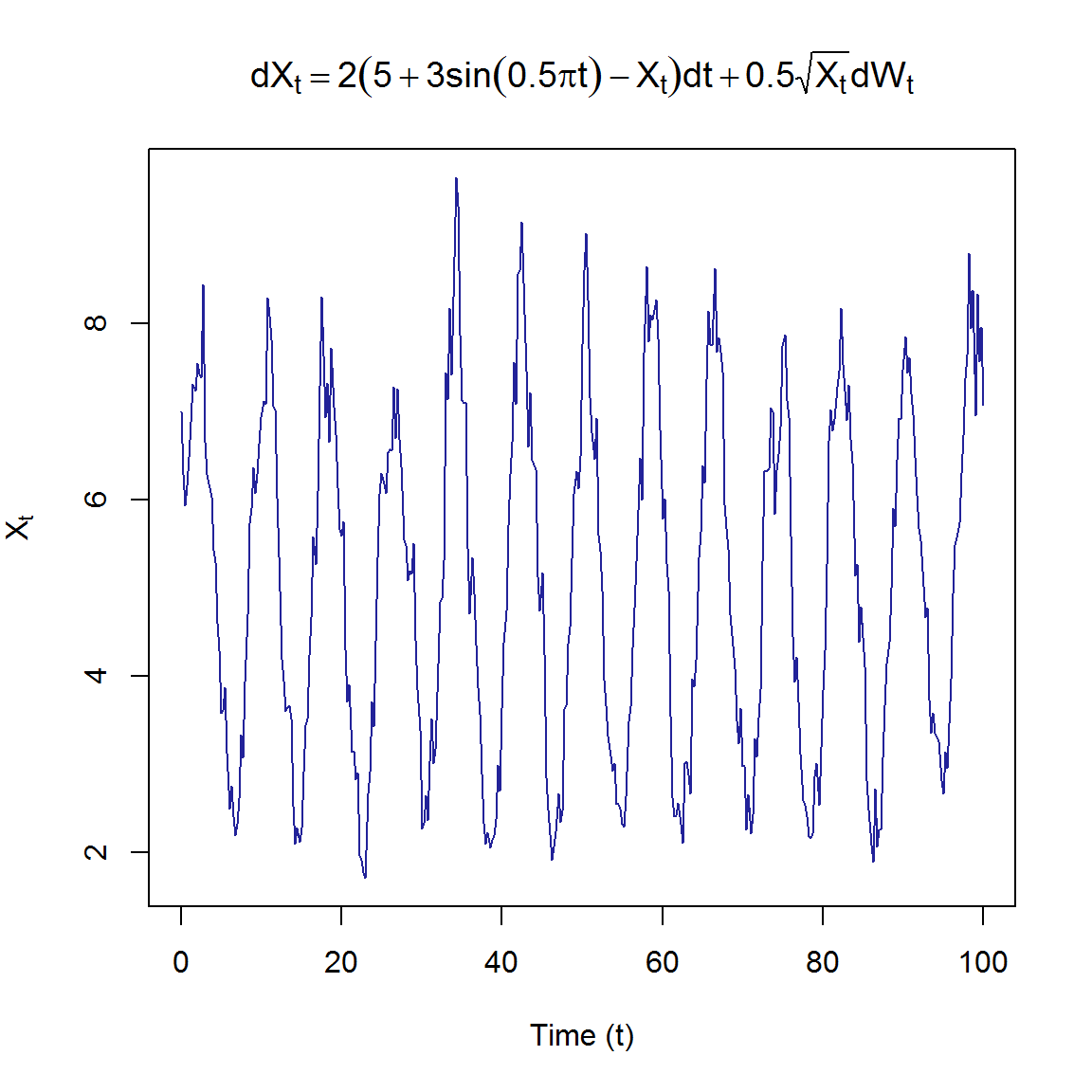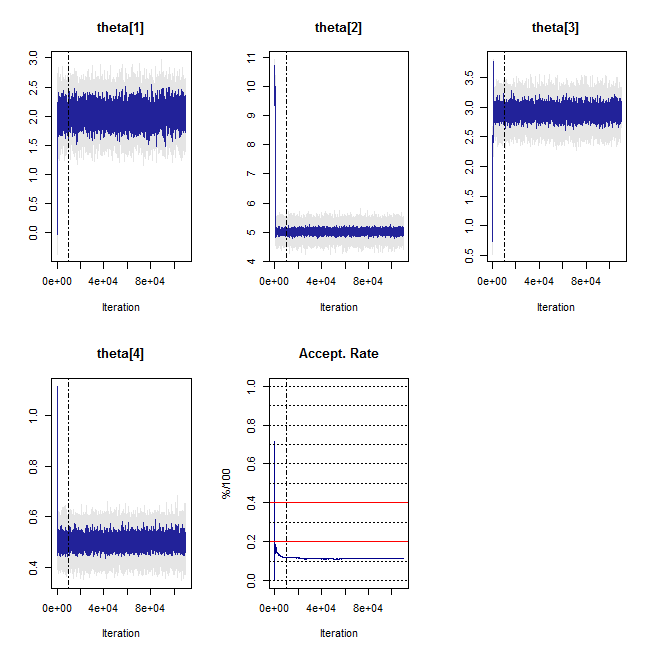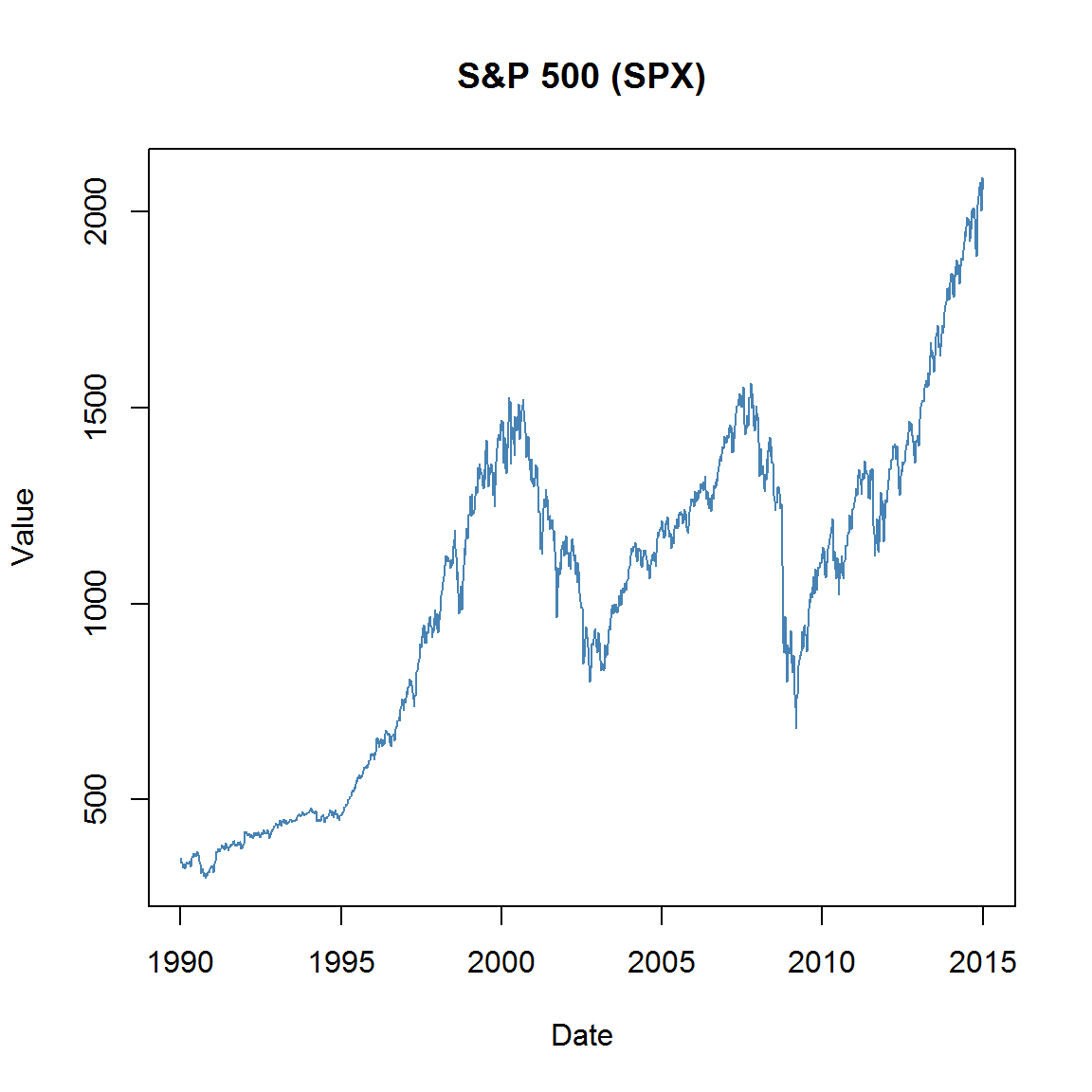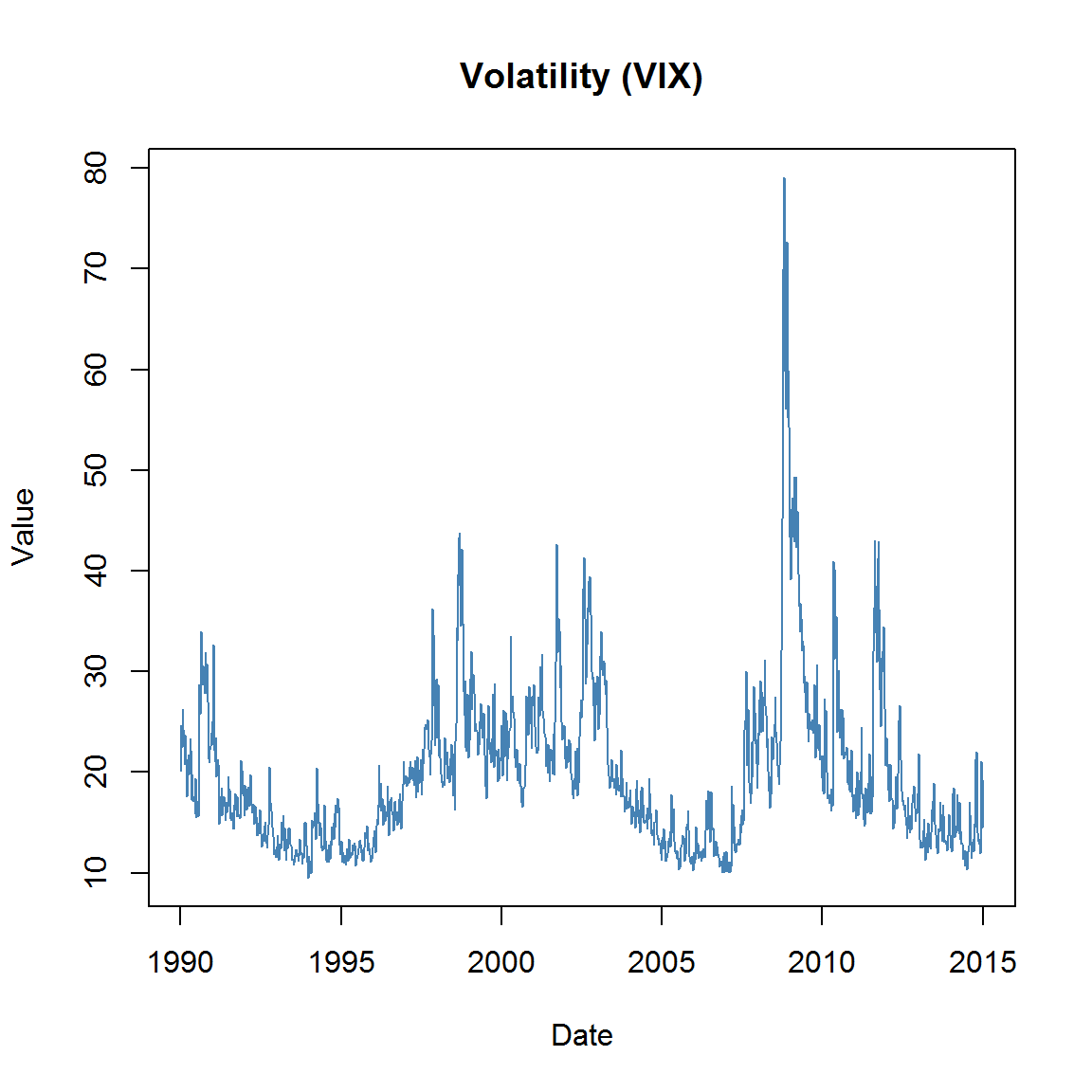# Likelihood based inference for discretely observed diffusion processes

Although partial differential equations (PDEs) with singular initial conditions such as the Kolmogorov forward equation which govern the transitional densities of diffusion processes present various difficulties from a computational perspective, the notion of a continuously evolving probability density function means that diffusion models can capture the dynamics of continuously evolving real-world phenomena in a very natural way. Although analytical solutions to the Kolmogorov equations can rarely be found, excellent methods for calculating analytical approximations of the transition density do exist. For example, Aït-Sahalia and others (2008) derive accurate short-horizon approximations to the Kolmogorv equations based on a Hermite series that can accommodate non-linearities in the drift and diffusion of the model. Huang (2011) applies Wagner-Platen expansions (see Platen (1999)) to the conditional moments of a target diffusion which can then be plugged into a surrogate density in order to yield a short-transition horizon approximation to the transition density. In order to push beyond the threshold of short-horizon approximations one has to resort to numerical methods. However, although numerical schemes such as the Crank-Nicolson method (Crank and Nicolson 1996) or the method of lines (MOL) (Hamdi, Schiesser, and Griffiths 2007) can be used to solve the Kolmogorov equations directly, the computational burden of such schemes may magnify significantly in the present context. This follows since calculating quantities of interest such as the likelihood for a discretely observed trajectory relies on numerous evaluations of the transitional density, possibly over varying time-scales. In combination these methods produce desirable results for a wide variety of non-linear diffusions, however, the aim of the present paper is to develop software that can handle inference at sample resolutions that may be too sparse for analytical short-horizon expansions whilst still remaining computationally feasible in a non-parallel computing environment. As such we adopt the cumulant truncation procedure (see the Vignette on transitional densities) developed by Varughese (2013), whereby the transition density can be approximated accurately and efficiently over arbitrarily large transition horizons for a suitably general class of non-linear diffusion models.

For purposes of inference we shall assume that the drift and diffusion terms - and consequently the transitional density - are dependent on a vector of parameters, $$\boldsymbol\theta$$. When a finite number of data points of the continuous process $$\mathbf{X}_t$$ is observed at discrete time epochs $$\{t_1,t_2,\ldots,t_N\}$$, resulting in the set of observations $$\{\mathbf{X}_{t_i}:i=1,\ldots,N\}$$, the Markov property of the diffusion $$X_t$$ can be used in order to represent the likelihood as a product of the transitional densities of the process (Aït-Sahalia et al. 2008): $L(\boldsymbol\theta|\{\mathbf{X}_{t_i}:i=1,\ldots,N\}) \propto \prod_{i=1}^{N-1} f(\mathbf{X}_{t_{i+1}}|\mathbf{X}_{t_{i}}).$ Thus, by approximating the transition density for a given diffusion model and plugging it into the likelihood equation, we may estimate the parameter vector $$\boldsymbol\theta$$ using standard likelihood-based inference techniques.

# Using DiffusionRgqd to perform inference on a diffusion model

The DiffusionRgqd package provides functions for efficiently calculating the likelihood of a discretely observed process trajectory under a diffusion model nested within the GQD-framework. This is achieved through the GQD.mle(), GQD.mcmc(), BiGQD.mle(), and BiGQD.mle() functions. Using the same interface as before (see for example the introductory vignette on generating transition densities), one can parametrize a diffusion model using the reserved variable name theta. For example, an Ornstein-Uhlenbeck model with SDE: $dX_t = \theta_1(\theta_2-X_t)dt+\sqrt{\theta_3^2}dB_t$ can be defined in R as:

G0=function(t){theta*theta}
G1=function(t){-theta}
Q0=function(t){theta*theta}

Subsequently, given a discretely observed trajectory along with a time index, one can calculate maximum likelihood estimates for the parameter vector theta. Consider for example the diffusion:

$dX_t = 2(5+3\sin(0.25 \pi t)-X_t)dt+0.5\sqrt{X_t}dB_t$

For convenience, we have included a simulated trajectory from the quadratic diffusion:

 library("DiffusionRgqd")
data(SDEsim1)
attach(SDEsim1)
par(mfrow=c(1,1))
expr1=expression(dX[t]==2*(5+3*sin(0.5*pi*t)-X[t])*dt+0.5*sqrt(X[t])*dW[t])
plot(SDEsim1$Xt~SDEsim1$time, type = 'l', col = '#222299', xlab = 'Time (t)', ylab = expression(X[t]), main = expr1)Using the GQD.mcmc() function, we can calculate parameter estimates under the simulated trajectory. For example:

GQD.remove()

G0 <- function(t){theta*(theta+theta*sin(0.25*pi*t))}
G1 <- function(t){-theta}
Q1 <- function(t){theta*theta}

theta   <- c(1, 10, 1, 1)               # Starting values for the chain
sds     <- c(0.25, 0.25, 0.2, 0.05)/1.5 # Std devs for proposal distributions
mesh    <- 10                           # Number of mesh points
burns   <- 10000                        # Burn 10000 updates

# Run the MCMC procedure for the model defined above:
model_1 <- GQD.mcmc(SDEsim1$Xt, SDEsim1$time, mesh, theta, sds, updates, burns)After running the desired number of updates, GQD.mcmc() will make a diagnostic plot in the form of a trace plot of the parameter vector and the acceptance rate of the MCMC chain. Subsequently we can calculate parameter estimates from the MCMC output using the GQD.estimates() function, which will also produce an ACF plot for the appropriately thinned parameter chain.

GQD.estimates(model_1, thin = 100, burns = 10000, corrmat = TRUE)$estimates Estimate Lower_CI Upper_CI theta 2.025 1.808 2.242 theta 5.017 4.917 5.109 theta 2.917 2.781 3.058 theta 0.501 0.472 0.533$corrmat
theta theta theta theta
theta     1.00    -0.19    -0.37     0.35
theta    -0.19     1.00     0.43    -0.04
theta    -0.37     0.43     1.00    -0.14
theta     0.35    -0.04    -0.14     1.00

# Maximum likelihood estimation: Stochastic volatility models

Diffusion processes are often used in financial applications to model the trajectories of asset prices or financial instruments. Although a great deal of literature is dedicated to the fitting and calibration of well known diffusion models to financial data, less time is spent assessing how well the dynamics of such models represent that which is observed in a given real-world dataset. Thus, for the present example we analyse a dataset that is often used to demonstrate the application of stochastic volatility models in finance, namely the Standard and Poor’s 500 index (SPX). Although the term ‘stochastic volatility’ may be used to refer to any model with randomly varying higher order moments, in the context of diffusion models it usually refers to the process of treating the volatility terms of a scalar diffusion model as being driven by a stochastic process itself. Perhaps the most famous stochastic volatility model is the Heston model (Heston 1993), a bivariate SDE of the form:

\begin{aligned} dS_t &= \theta_1S_tdt +\theta_2S_t\sqrt{V_t}dB_t^{(1)}\\ dV_t &= (\theta_3-\theta_4V_t)dt +\theta_6dB_t^{(2)}\\ \end{aligned}

where St denotes the spot price process and Vt denotes the corresponding variance process. Thus, in the Heston model the spot price is modelled by a geometric Brownian motion with the addition that the volatility coefficient for the spot price is itself driven by a diffusion process – in this case, a CIR process. Furthermore, it is assumed that the Brownian motion $$B_t^{(1)}$$ and $$B_t^{(2)}$$ are correlated. That is:

$\mbox{corr}(B_t^{(1)},B_t^{(2)}) = \theta_6.$

For the SPX, although data for the spot process $$S_t$$ is readily available, obtaining the trajectory of the volatility process is less trivial. In order to conduct the analysis we shall assume a suitable proxy for the volatility of the index by making use if the Chicago Board Options Exchange (CBOE) Market Volatility Index (VIX), which is based on the implied volatility of options written on the SPX. We source data for the index by using the Quandl package as follows:

library(Quandl)
## Loading required package: xts
## Loading required package: zoo
##
## Attaching package: 'zoo'
## The following objects are masked from 'package:base':
##
##     as.Date, as.Date.numeric
library(DiffusionRgqd)
# Source data for the S&P500 index (SPX).
quandldata1 <- Quandl("YAHOO/INDEX_GSPC", collapse = "weekly",
start_date = "1990-01-01", end_date = "2015-01-01", type = "raw")
St <- rev(quandldata1[,names(quandldata1)=='Close'])
time1 <-rev(quandldata1[,names(quandldata1)=='Date'])
# Source data for the volatility index (VIX).
quandldata2 <- Quandl("YAHOO/INDEX_VIX", collapse = "weekly",
start_date = "1990-01-01", end_date = "2015-01-01", type = "raw")
Vt <- rev(quandldata2[,names(quandldata2)=='Close'])
time2 <- rev(quandldata2[,names(quandldata2)=='Date'])

plot(St~time1, type= 'l', col ='steelblue', main = 'S&P 500 (SPX)', ylab  = 'Value', xlab = 'Date')plot(Vt~time1, type= 'l', col ='steelblue', main = 'Volatility (VIX)', ylab  = 'Value', xlab = 'Date')The resulting figure plots the resulting weekly closing prices for the SPX and VIX for the period 1990-01-01 to 2015-01-01. Visual inspection of the time series suggests that the volatility of the index does indeed vary significantly over time as is evidenced by the VIX which was observed to be as low as 9.5% during December of 1993 and high as 79% during October of 2008. In order to conduct the analysis we consider the log-returns of the index. Let $$R_t = log(S_t)$$ then by Ito’s lemma, the corresponding Heston model under the log-transform is given by:

\begin{aligned} dS_t &= (\theta_1 - 0.5\theta_2^2V_t)dt +\theta_2\sqrt{V_t}dB_t^{(1)}\\ dV_t &= (\theta_3-\theta_4V_t)dt +\theta_6dB_t^{(2)}\\ \end{aligned}

In order to incorporate the correlation coefficient of the Brownian motions into the SDE it is useful to write the SDE in terms of independent Brownian motions: Let $$W_t^{(1)}$$ and $$W_t^{(2)}$$ be independent Brownian motions then we can construct correlated Brownian motions $$B_t^{(1)}$$ and $$B_t^{(2)}$$ with $$\mbox{corr}(B_t^{(1)},B_t^{(2)}) = \theta_6$$ through the standard translation:

$\begin{bmatrix} dB_t^{(1)}\\ dB_t^{(2)}\\ \end{bmatrix} = \begin{bmatrix} 1&0\\ \theta_6&\sqrt{1-\theta_6^2}\\ \end{bmatrix} \begin{bmatrix} dW_t^{(1)}\\ dW_t^{(2)}\\ \end{bmatrix}.$ Thus the appropriate diffusion tensor for the Heston model is given by: $\begin{bmatrix} \theta_2\sqrt{V_t}&0\\ 0&\theta_5\sqrt{V_t}\\ \end{bmatrix} \begin{bmatrix} 1&\theta_6\\ \theta_6&1\\ \end{bmatrix} \begin{bmatrix} \theta_2\sqrt{V_t}&0\\ 0&\theta_5\sqrt{V_t}\\ \end{bmatrix}^\prime = \begin{bmatrix} \theta_2^2V_t&\theta_2\theta_5\theta_6V_t\\ \theta_2\theta_5\theta_6V_t&\theta_5^2V_t\\ \end{bmatrix}.$

Now, within the generalized quadratic framework, the bivariate template diffusion model is of the form: $d\mathbf{X}_t =\boldsymbol\mu(\mathbf{X}_t,t)dt+\boldsymbol\sigma(\mathbf{X}_t,t)d\mathbf{B}_t$ where

$\boldsymbol\mu(\mathbf{X}_t,t)= \begin{bmatrix} \sum_{i+j\leq 2}a_{ij}(t)X_t^iY_t^j\\ \sum_{i+j\leq 2}b_{ij}(t)X_t^iY_t^j\\ \end{bmatrix}$

and

$\boldsymbol\sigma(\mathbf{X}_t,t)\boldsymbol\sigma^{\prime}(\mathbf{X}_t,t) = \begin{bmatrix} \sum_{i+j\leq 2}c_{ij}(t)X_t^iY_t^j&\sum_{i+j\leq 2}d_{ij}(t)X_t^iY_t^j\\ \sum_{i+j\leq 2}e_{ij}(t)X_t^iY_t^j&\sum_{i+j\leq 2}f_{ij}(t)X_t^iY_t^j\\ \end{bmatrix}.$ Thus, the Heston model can be fitted using the R code:

GQD.remove() # Remove the previous model coefficients
##  "Removed :  G0 G1 Q0"
# R_t coefficients:
a00 <- function(t){theta}
a01 <- function(t){-0.5*theta*theta}
c01 <- function(t){theta*theta}
d01 <- function(t){theta*theta*theta}
# V_t coefficients:
b00 <- function(t){theta}
b01 <- function(t){-theta}
e01 <- function(t){theta*theta*theta}
f01 <- function(t){theta*theta}

# Create data matrix and numerical time vector :
X <- cbind(log(St),(Vt/100)^2)
time <- cumsum(c(0, diff(as.Date(time1))*(1/365)))

# Some starting parameters for the optimization routine:
theta.start <- c(0, 1, 1, 0.5, 1, 0)
# Calculate MLEs of the parameter vector:
model_1 <- BiGQD.mle(X, time, mesh = 10, theta = theta.start)
## Warning in BiGQD.mle(X, time, mesh = 10, theta = theta.start):
## ==============================================================================
## 39. Input: Time series contains values of small magnitude.
##     This may result in numerical instabilities.
##     It may be advisable to scale the data by a constant factor.
## ==============================================================================
## Compiling C++ code. Please wait.

##  ================================================================
##  ================================================================
##  _____________________ Drift Coefficients _______________________
##  a00 : theta
##  a01 : -0.5*theta*theta
##  ...   ...   ...   ...   ...   ...   ...   ...   ...   ...   ...
##  b00 : theta
##  b01 : -theta
##  ___________________ Diffusion Coefficients _____________________
##  c01 : theta*theta
##  ...   ...   ...   ...   ...   ...   ...   ...   ...   ...   ...
##  d01 : theta*theta*theta
##  ...   ...   ...   ...   ...   ...   ...   ...   ...   ...   ...
##  e01 : theta*theta*theta
##  ...   ...   ...   ...   ...   ...   ...   ...   ...   ...   ...
##  f01 : theta*theta
##
##  __________________________ Model Info __________________________
##  Time Homogeneous    : Yes
##  Data Resolution     : Homogeneous: dt=0.0192
##  # Removed Transits. : None
##  Density approx.     : 4th Ord. Truncation, Bivariate-Saddlepoint
##  Elapsed time        : 00:00:19
##  ...   ...   ...   ...   ...   ...   ...   ...   ...   ...   ...
##  dim(theta)          : 6
##  ----------------------------------------------------------------
# Retreve parameter estimates and appr. 95% CIs:
GQD.estimates(model_1)
##          Estimate Lower_95 Upper_95
## theta    0.083    0.031    0.135
## theta    0.770    0.740    0.799
## theta    0.167    0.128    0.207
## theta    3.819    2.813    4.824
## theta    0.431    0.414    0.447
## theta   -0.671   -0.700   -0.641

The Heston model thus provides insight into the dynamics of the SPX and its corresponding variance dynamics. Indeed, the resulting parameter estimates confirms well documented features such as strong negative correlation in the noise of the SPX and VIX series as evidenced by the correlation parameter $$\theta_6$$. By modifying the Heston model we can easily perform a more detailed analysis by including other peripheral data such as risk free rates and dividends in order to formulate the model in the market context (see for example Hurn, Lindsay, and McClelland (2015)).

browseVignettes('DiffusionRgqd')